# Changing the Sign for Aggregation## Use Case:

In a lot of financial account hierarchies, costs are shown as positive numbers—but need to be subtracted from the totals.  With Anaplan, this is easily achievable with line items.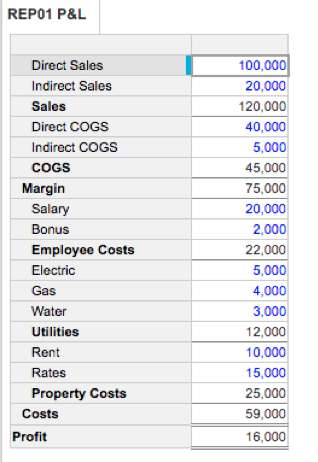The line item formulae are as follows:

• Margin = Sales – COGS
• Profit = Margin – Costs

This is a very simple example that is easily solved.  However, often there are additional calculations that need to be performed on the data—meaning the financial accounts are represented by a list.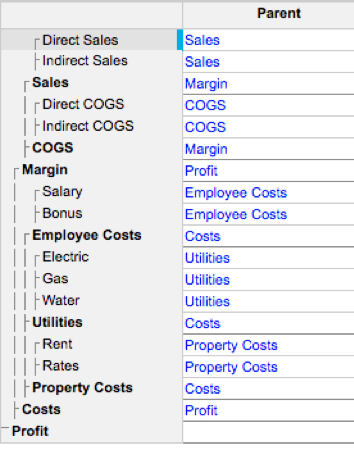As lists work on straight aggregation, the same data from above does not calculate in the way we require.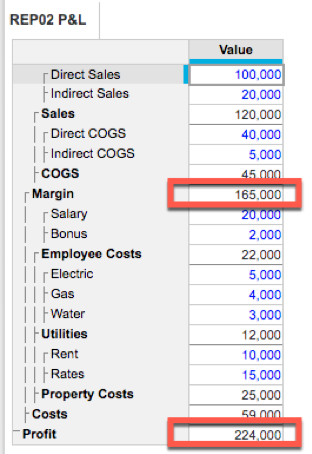## Solution:

It requires the use of two additional line items and the use of the ratio summary method.

1. Create a P&L Signage module.

• Dimension this by the P&L list.
• Add line items to hold the signage.

In the example below, I have used a Boolean to signify which lines should be “flipped”, although you could just use the signage line item and manually enter the values.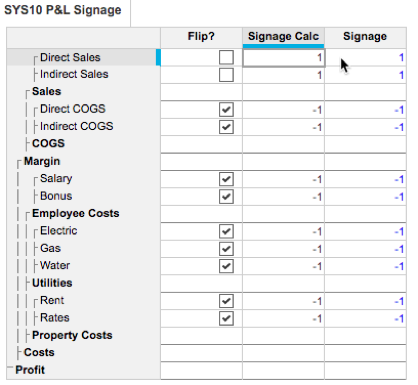2. In the P&L Module:

a. Create two additional line items

• Temp
• Ratio

Note: The ratio line item only needs to be dimensioned by the P&L list, so this is likely to be a subsidiary view.

• Temp = Value * Ratio
• Ratio = 'SYS10 P&L Signage'.Signage Calc

3. Amend the summary option for the Value line item to Ratio as follows: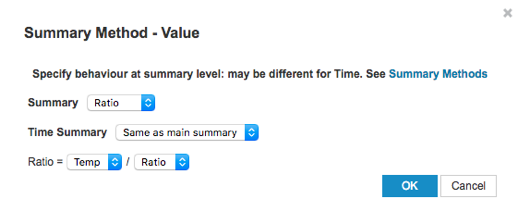4. Amend the summary option for the Ratio line item to Max.

This last setting is the key to getting the totals to work correctly.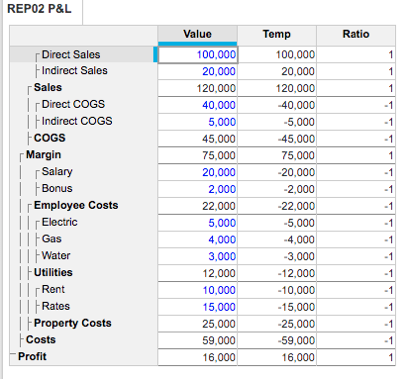This will also work if the costs are “credits”, so it should be shown as negative values.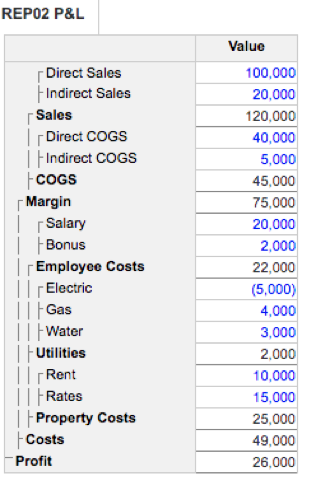It also works if all the costs within a subtotal are credits.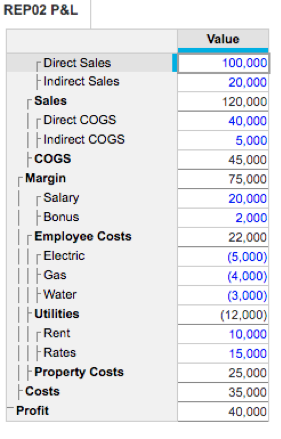The final example is if you have a time dimension in the module (which is very likely needed).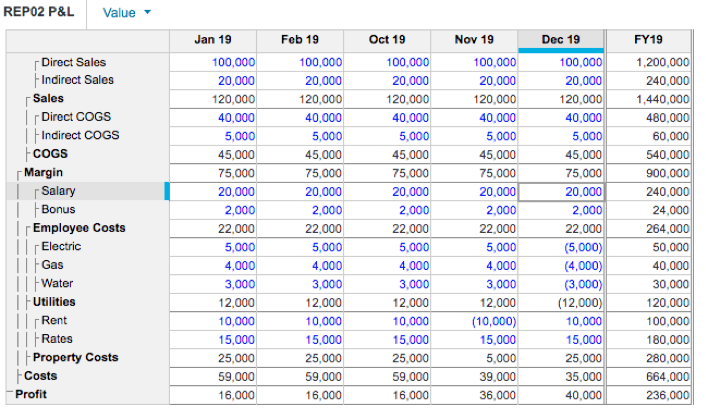If you are using a line item subset as a dimension of a module, this technique, using ratio, is particularly relevant because line item subsets require the totals to be simple aggregation in the same was as a list does.

To achieve the same result, follow the steps above, but your modules will have the line item subset as the dimension instead of the P&L list.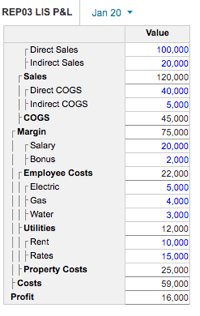Tagged:

•Brilliant stuff David. You never cease to amaze! This piece of functionality will allow users to keep the reporting signage, but be able to have a proper aggregating report

•Yeah, I wish this had been documented (or I would have come up with this solution) three years ago when I had to **** my head against the wall and add a ton of temporary line items to get this type of reporting implemented for a client of mine.

•David, thanks for the nice solution summary. We have a trade off here that the breakback function is no longer working with ratio (vs. sum) summary type. Would be nice to have both working😂